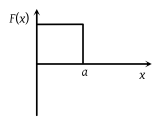You’ve reached the end of your free Videos limit.
#5 | Potential Energy
(Physics) > Work,Energy and Power
Related Practice Questions :

The potential energy of a body is given by, U = ABx2 (Where x is the displacement). The magnitude of force acting on the particle is

(1) Constant

(2) Proportional to x

(3) Proportional to x2

(4) Inversely proportional to x

Complete Question Bank + Test Series
Complete Question Bank

Difficulty Level:

The potential energy between two atoms in a molecule is given by $U\left(x\right)=\frac{a}{{x}^{12}}-\frac{b}{{x}^{6}}$; where a and b are positive constants and x is the distance between the atoms. The atoms are in stable equilibrium when:

(1) $x=\sqrt{\frac{11a}{5b}}$

(2) $x=\sqrt{\frac{a}{2b}}$

(3) x = 0

(4) $x=\sqrt{\frac{2a}{b}}$

Complete Question Bank + Test Series
Complete Question Bank

Difficulty Level:

A particle of mass m is moving in a horizontal circle of radius r under a centripetal force equal to –K/r2, where K is a constant. The total energy of the particle is

(1) $\frac{K}{2r}$

(2) $-\frac{K}{2r}$

(3) $-\frac{K}{r}$

(4) $\frac{K}{r}$

Complete Question Bank + Test Series
Complete Question Bank

Difficulty Level:

A particle free to move along the x-axis has potential energy given by $U\left(x\right)=k\left[1-{e}^{{\left(-x\right)}^{2}}\right]$ for $-\infty \le x\le +\infty$, where k is a positive constant of appropriate dimensions. Then

(1) At point away from the origin, the particle is in unstable equilibrium

(2) For any finite non-zero value of x, there is a force directed away from the origin

(3) If its total mechanical energy is k/2, it has its minimum kinetic energy at the origin

(4) For small displacements from x = 0, the motion is simple harmonic

Complete Question Bank + Test Series
Complete Question Bank

Difficulty Level:

The potential energy of a system is represented in the first figure. the force acting on the system will be represented by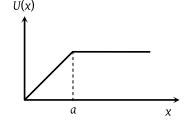(1)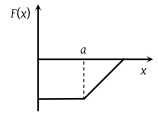(2)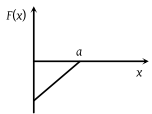(3)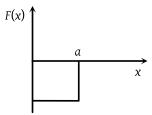(4)